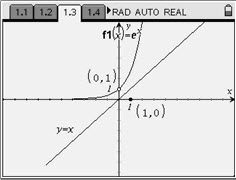# Activities

••• ##### Subject Area

• Math: Algebra II: Logarithms and Exponentials
• Math: Algebra II: Inverses
• Math: Algebra II: Logarithmic Functions

• ##### Author9-12

15 Minutes

• ##### Device
• TI-Nspire™
• TI-Nspire™ CAS
• ##### Software

TI-Nspire™
TI-Nspire™ CAS

1.7

## Exponential Reflections#### Activity Overview

In this activity, students will reflect a point from the exponential function over the line y = x and find the locus of the reflection, which is the natural log function.

#### Key Steps

•Students begin by reflecting the given point over the line y = x. To do this, select MENU > Transformation > Reflection, then select the point followed by the line y = x. Then, use MENU > Actions > Coordinates and Equations to get the coordinates of the reflected point. Students should record the new point in the table. Have students change the value of the point at (0, 1) by changing the x-value. Double-click on the x-value and type in the new values from the table.